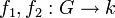# Inner product of functions

## Definition

### Bilinear form

This definition works in all non-modular characteristics and is bilinear.

Let$G$ be a finite group and$k$ be a field whose characteristic does not divide the order of$G$. Given two functions$f_1,f_2:G \to k$, define:$\langle f_1, f_2 \rangle_G = \frac{1}{|G|} \sum_{g \in G} f_1(g)f_2(g^{-1})$

Note that$1/|G|$ makes sense as an element of$k$ because$G$ is finite and the characteristic of$k$ does not divide the order of$G$.

### Hermitian inner product

This definition works over$\mathbb{C}$ or any subfield of$\mathbb{C}$ that is closed under complex conjugation.

Let$k$ be such a field and$G$ be a finite group. Given two functions$f_1,f_2:G \to k$, define:$\langle f_1, f_2 \rangle_G = \frac{1}{|G|} \sum_{g \in G} f_1(g)\overline{f_2(g)}$

Note that this is a Hermitian positive-definite inner product.

### Relation between the definitions

First, note that the two inner products defined are not the same thing over a field where both definitions are applicable. The former is bilinear while the latter is sesquilinear and positive-definite (these qualities make it Hermitian). However, the following is true:

For a character$\chi$ of a representation of a finite group$G$ and an element$g \in G$,$\chi(g^{-1}) = \overline{\chi(g)}$. See trace of inverse is complex conjugate of trace.

Thus, if we apply the inner product operation only to characters of representations, then both definitions work exactly the same way.